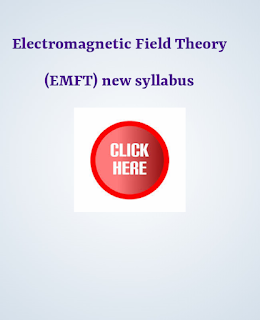# Aku EEE 3rd semester  Electromagnetic Field theory (EMFT) free full notes /Electromagnetic Field theory (EMFT) full notes according to new syllabus /Aku EEE 3rd semester   Electromagnetic Field ( EMFT) theory new syllabusAku EEE 3rd semester  Electromagnetic Field theory (EMFT) full notes/Electromagnetic Field theory (EMFT) full notes according to new syllabus /Aku EEE 3rd semester   Electromagnetic Field ( EMFT) theory new syllabus

### Aku EEE 3rd semester   Electromagnetic Field ( EMFT) theory new syllabusAku EEE 3rd semester  Electromagnetic Field theory (EMFT) full notes /Electromagnetic Field theory (EMFT) full notes according to new syllabus /Aku EEE 3rd semester   Electromagnetic Field ( EMFT) theory new syllabus

#### Module 1: Review of Vector Calculus (6 hours)

Vector algebra-addition, subtraction, components of vectors, scalar and vector multiplications, triple products, three orthogonal coordinate systems (rectangular, cylindrical and spherical). Vector calculus-differentiation, partial differentiation, integration, vector operator del, gradient, divergence a n d curl; integral theorems of vectors. Conversion of a vector from one coordinate system to an other.

#### Module 2: Static Electric Field (6 Hours)

Coulomb’s law, Electric field intensity, Electrical field due to point charges. Line, Surface and Volume charge distributions. Gauss law and its applications. Absolute Electric potential, Potential difference, Calculation of potential differences for different configurations. Electric dipole, Electrostatic Energy and Energy density.

#### Module 3: Conductors, Dielectrics and Capacitance (6 Hours)

Current and current density, Ohms Law in Point form, Continuity of current, Boundary conditions of perfect dielectric materials. Permittivity of dielectric materials, Capacitance, Capacitance of a two wire line, Poisson’s equation, Laplace’s equation, Solution of Laplace and Poisson’s equation, Application of Laplace’s and Poisson’s equations.

#### Module 4: Static Magnetic Fields (5 Hours)

Biot-Savart Law, Ampere Law, Magnetic flux and magnetic flux density, Scalar and Vector Magnetic potentials. Steady magnetic fields produced by current carrying conductors.

#### Module 5: Magnetic Forces, Materials and Inductance (6 Hours)

Force on a moving charge, Force on a differential current element, Force between differential current elements, Nature of magnetic materials, Magnetization and permeability, Magnetic boundary conditions, Magnetic circuits, inductances and mutual inductances.

#### Module 6: Time Varying Fields and Maxwell’s Equations (5 Hours)

Faraday’s law for Electromagnetic induction, Displacement current, Point form of Maxwell’s equation, Integral form of Maxwell’s equations, Motional Electromotive forces. Boundary Conditions.

#### Module 7: Electromagnetic Waves (6 Hours)

Derivation of Wave Equation, Uniform Plane Waves, Maxwell’s equation in Phasor form, Wave equation in Phasor form, Plane waves in free space and in a homogenous material. Wave equation for a conducting medium, Plane waves in lossy dielectrics, Propagation in good conductors, Skin effect. Poynting theorem.

#### Module 8: Transmission line (4 Hours)

Introduction, Concept of distributed elements, Equations of voltage and current, Standing waves and impedance transformation, Lossless and low-loss transmission lines, Power transfer on a transmission line, Analysis of transmission line in terms of admittances, Transmission line calculations with the help of Smith chart, Applications of transmission line, Impedance matching using transmission lines.

"Aku EEE 3rd semester  Electromagnetic Field theory (EMFT) full notes /Electromagnetic Field theory (EMFT) full notes according to new syllabus /Aku EEE 3rd semester   Electromagnetic Field ( EMFT) theory new syllabus"

#### Text/References:

1. M. N. O. Sadiku, “Elements of Electromagnetics”, Oxford University Publication, 2014.
2. A. Pramanik, “Electromagnetism - Theory and applications”, PHI Learning Pvt. Ltd, New Delhi, 2009.
3. A. Pramanik, “Electromagnetism-Problems with solution”, Prentice Hall India, 2012.
4. G.W. Carter, “The electromagnetic field in its engineering aspects”, Longmans, 1954.
5. W.J. Duffin, “Electricity and Magnetism”, McGraw Hill Publication, 1980.
6. W.J. Duffin, “Advanced Electricity and Magnetism”, McGraw Hill, 1968.
7. E.G. Cullwick, “The Fundamentals of Electromagnetism”, Cambridge University Press, 1966.
8. B. D. Popovic, “Introductory Engineering Electromagnetics”, Addison-Wesley Educational Publishers, International Edition, 1971.
9. W. Hayt, “Engineering Electromagnetics”, McGraw Hill Education, 2012.

"Aku EEE 3rd semester  Electromagnetic Field theory notes (EMFT)/Electromagnetic Field theory (EMFT) full notes according to new syllabus /Aku EEE 3rd semester   Electromagnetic Field ( EMFT) theory new syllabus"Aku EEE 3rd semester  Electromagnetic Field theory (EMFT) free full notes /Electromagnetic Field theory (EMFT) full notes according to new syllabus /Aku EEE 3rd semester   Electromagnetic Field ( EMFT) theory new syllabus